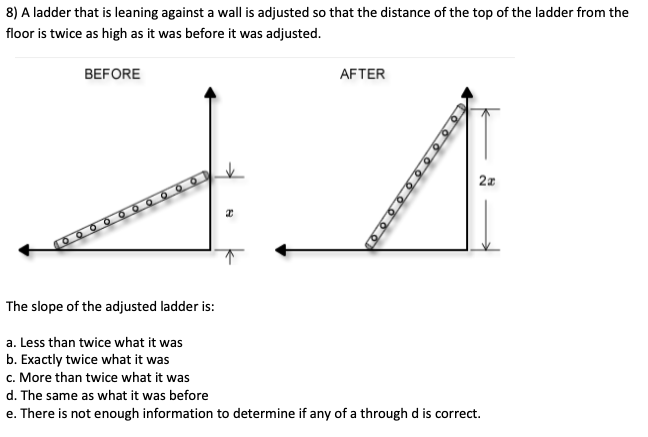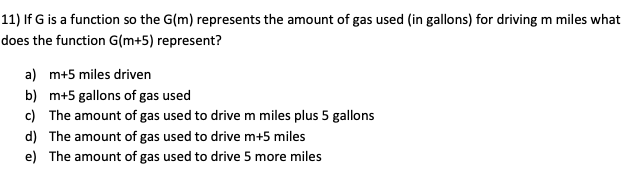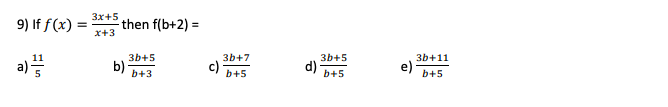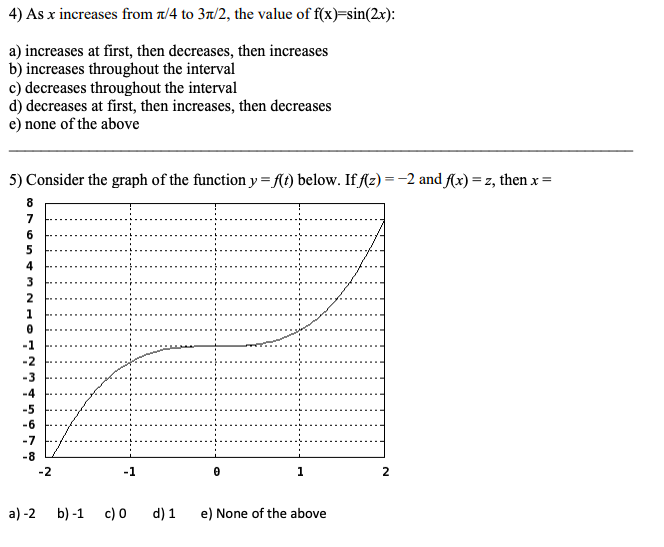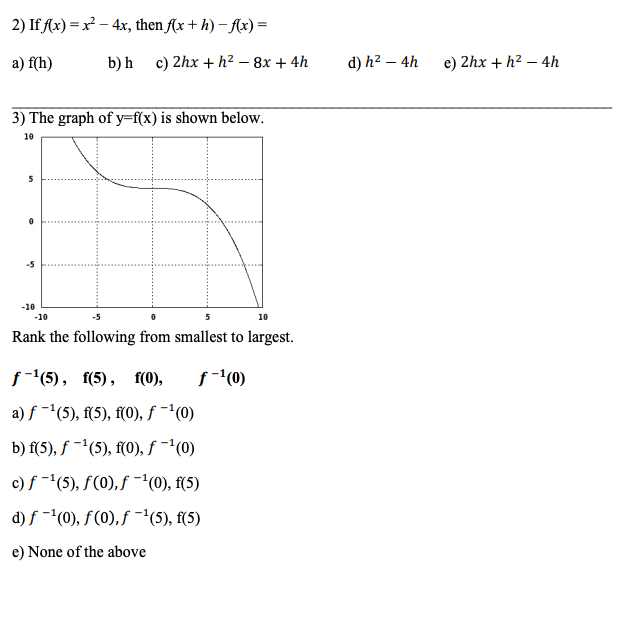Transcribed Image Text: 8) A ladder that is leaning against a wall is adjusted so that the distance of the top of the ladder from the floor is twice as high as it was before it was adjusted. BEFORE AFTER ZA OOOOOOOOOO The slope of the adjusted ladder is: a. Less than twice what it was b. Exactly twice what it was c. More than twice what it was d. The same as what it was before e. There is not enough information to determine if any of a through d is correct. 11) If G is a function so the G(m) represents the amount of gas used in gallons) for driving m miles what does the function (m+5) represent? a) m+5 miles driven b) m+5 gallons of gas used c) The amount of gas used to drive m miles plus 5 gallons d) The amount of gas used to drive m+5 miles e) The amount of gas used to drive 5 more miles 9) if f(x) = * then f(b+2) = 4) As x increases from 1/4 to 31/2, the value of f(x)=sin(2x): a) increases at first, then decreases, then increases b) increases throughout the interval c) decreases throughout the interval d) decreases at first, then increases, then decreases e) none of the above 5) Consider the graph of the function y = f(t) below. If f(2)=-2 and f(x)=z, then x = o ou UNOPNWU a)-2 b)-1 c) d)1 e) None of the above 2) If f(x) = x2 - 4x, then f(x+h)-f(x) = a) f(h) b)h c) 2x + h2 – 8x + 4h d) h2 – 4h e) 2x + h2 – 4h 3) The graph of y=f(x) is shown below. -10 -10 -5 Rank the following from smallest to largest. f-15), f(5), f(0), f-10) a) f-4(5), f(5), f(o), f -(0) b) f(5), f -(5), f(0), f - (0) c) f -(5), f(0,f-0), f(5) d) f-0), f(0,f-(5), f(5) e) None of the above
More
Transcribed Image Text: 8) A ladder that is leaning against a wall is adjusted so that the distance of the top of the ladder from the floor is twice as high as it was before it was adjusted. BEFORE AFTER ZA OOOOOOOOOO The slope of the adjusted ladder is: a. Less than twice what it was b. Exactly twice what it was c. More than twice what it was d. The same as what it was before e. There is not enough information to determine if any of a through d is correct. 11) If G is a function so the G(m) represents the amount of gas used in gallons) for driving m miles what does the function (m+5) represent? a) m+5 miles driven b) m+5 gallons of gas used c) The amount of gas used to drive m miles plus 5 gallons d) The amount of gas used to drive m+5 miles e) The amount of gas used to drive 5 more miles 9) if f(x) = * then f(b+2) = 4) As x increases from 1/4 to 31/2, the value of f(x)=sin(2x): a) increases at first, then decreases, then increases b) increases throughout the interval c) decreases throughout the interval d) decreases at first, then increases, then decreases e) none of the above 5) Consider the graph of the function y = f(t) below. If f(2)=-2 and f(x)=z, then x = o ou UNOPNWU a)-2 b)-1 c) d)1 e) None of the above 2) If f(x) = x2 - 4x, then f(x+h)-f(x) = a) f(h) b)h c) 2x + h2 – 8x + 4h d) h2 – 4h e) 2x + h2 – 4h 3) The graph of y=f(x) is shown below. -10 -10 -5 Rank the following from smallest to largest. f-15), f(5), f(0), f-10) a) f-4(5), f(5), f(o), f -(0) b) f(5), f -(5), f(0), f - (0) c) f -(5), f(0,f-0), f(5) d) f-0), f(0,f-(5), f(5) e) None of the above